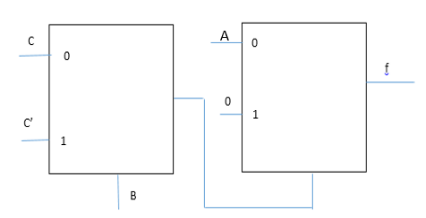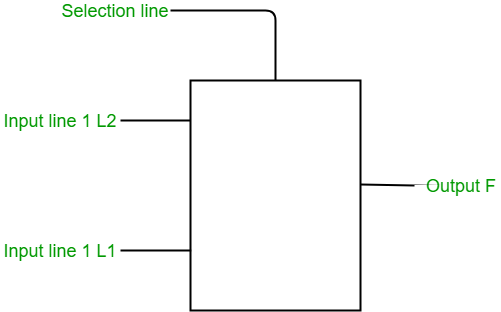# GATE | GATE MOCK 2017 | Question 16

• Last Updated : 19 Nov, 2018

The Boolean function f implemented in the figure shown below, using two input multiplexers is:(A) AB’C + ABC’

(B) A’B’C + A’BC’

(C) A’BC + A’B’C’

(D) ABC + AB’C’

Explanation: The output of a multiplexer (2X1) can be expressed in the form of:f= S’. L1 + S. L2

For the above diagram the output from the first multiplexer would be B’C + BC’ which acts a select line for the second multiplexer. Hence,
f= (B’C + BC’)’A + (B’C + BC’).0

= ((B’C)’ . (BC’)’)A + 0
= (B+C’)(B’ + C)A

= (BC + B’C’) A
= ABC + AB’C’

Quiz of this Question

My Personal Notes arrow_drop_up